# 1. 神经网络入门

## 1.1. Multi-Layer Neural Network

A. 3-layer network: Input Layer,Hidden Lyer,Output layer. Except input units,each unit has a bias.

### 1.1.1. preassumption calculation

\begin{aligned} net_{j} = \sum_{i=1}^{d}x_{i}w_{ji}+w_{j0}=\sum_{i=0}^{d}x_{i}w_{ji}=w_{j}^{t}x \end{aligned}

Specifically, a signal $x_{i}$ at the input of synapse $i$ connected to nueron $j$ us multiplied by the synaptic weight $w_{ji}$. $i$ refers input layer,$j$ refers hidden layer.$w_{j0}$ is the bias.$x_{0}=+1$.

• Each neuron is represented by a set of linear synaptic links, an externally applied bias, and a possibly nonlinear activation link.The bias is represented by a synaptic link connected to an input fixed at $+1$.
• The synaptic links of a neuron weight their respective input signals.
• The weighted sum of the input signals defines the induced local field of the neuron in question.
• The activation link squashes the induced local field of the neuron to produce an output. Output layer:

\begin{aligned} y_{j}=f(net_{j}) \end{aligned}

$f()$ is the activation function.It defines the output of a neuron in terms of the induced local field $net$ .

{% math %}
\begin{matrix}
x_{0}=+1 \ar[ddr]|(0.6){w_{j0}} &  &  \\
x_{1} \ar[r]|(0.6){w_{j1}} & B & C \\
x_{2} \ar[r]^(0.6){w_{j2}} & net_{j} \ar[r]^(0.6){f()} & y_{j} \\
x
\end{matrix}
{% endmath %}


For example: \begin{aligned} net_{k}=\sum_{j=1}^{n_{H}}y_{i}w_{kj}+w_{k0}=\sum_{j=0}^{n_{H}}x_{i}w_{ji}=w_{k}^{t}y \end{aligned} $n_{H}$is the number of hidden layers.

So: \begin{aligned} g_{k}(x)=f(\sum_{j=1}^{n_{H}}w_{kj}+f(\sum_{i=0}^{d}x_{i}w_{ji}+w_{j0})+w_{k0}) \end{aligned} The activate function of output layer can be different from hidden layer while each unit can have different activate function.

%% BP Algorithm %%

### 1.1.2. BP Algorithm

The popularity of on-line learning for the supervised training of multilayer perceptrons has been further enhanced by the development of the back-propagation algorithm. Backpropagation, an abbreviation for "backward propagation of errors",is the easiest way of supervised training.We need to generate output activations of each hidden layer. The partial derivative $\partial J /\partial w_{ji}$ represents a sensitivity factor, determining the direction of search in weight space for the synaptic weight $w_{ji}$. Learning: \begin{aligned} \mathcal T =\{ x(n),d(n)\}_{n=1}^{N}\\ e_{j}(n)=d_{j}(n)-y_{j}(n) \end{aligned} the instantaneous error energy of neuron $j$ is defined by \begin{aligned} J(w)=\frac 12 \sum_{k=1}^{c}(e_{k})^{2}=\frac 12||t-\delta||^{2} \\ \end{aligned} In the batch method of supervised learning, adjustments to the synaptic weights of the multilayer perceptron are performed \emph{after} the presentation of all the $N$ examples in the training sample $\mathcal T$ that constitute one \emph{epoch} of training. In other words, the cost function for batch learning is defined by the average error energy $J(w)$.

• firstly define the training bias of output layer: \begin{aligned} \Delta w=-\eta\frac {\partial J(w)}{\partial w} \\ w(m+1)=w(m)+/Delta w(m) \end{aligned}

\begin{aligned} \frac {\partial J}{\partial w_{kj}}=\frac {\partial J}{\partial net_{k}}\frac {\partial net_{k}}{\partial w_{kj}} \\ \frac {\partial J}{\partial net_{k}}= \frac {\partial J}{\partial \delta _{k}}\frac {\partial \delta _{k}}{\partial J}=-(t_{k}-\delta _{k})f'(net_{k}) \\ \Delta w_{kj}=\eta \frac {\partial J}{\partial net_{k}}=\eta (t_{k}-\delta _{k}))f'(net_{k})y_{j} \end{aligned}

• input->hidden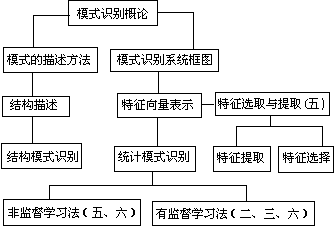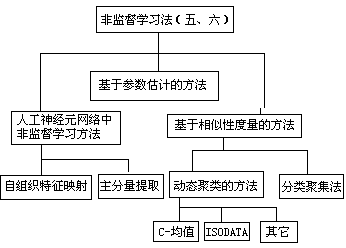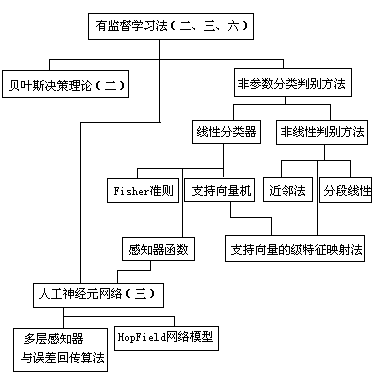High dimension：

• SVM ,拟合能力随维度增加线性增长
• kenel-based,内积
• 易收敛
• convex
• low computational cost

Going deep：神经网络，拟合能力随深度增加指数增长

• 复杂boundary
• non-convex
• Black-magic
• overfitting
• High computational cost
• 矩阵联乘

1.神经网络获取的知识是从外界环境中学习得来的； 2.互连神经元的连接强度，即突触权值，用于储存获取的知识。

# 2. 神经元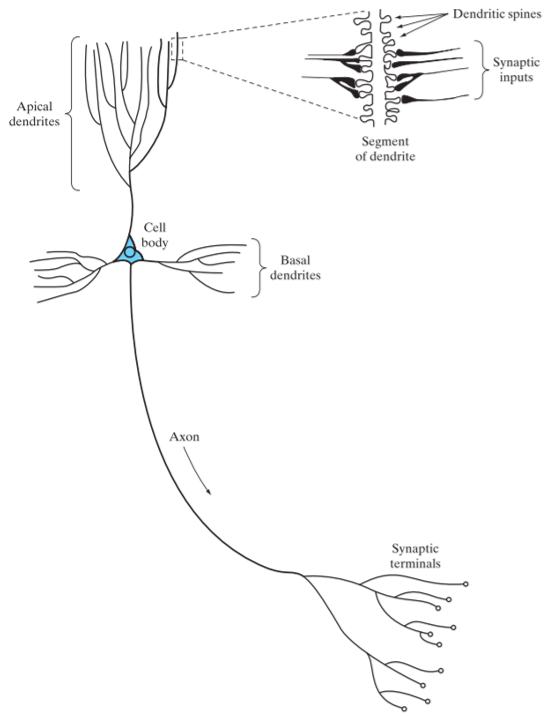## 2.1. 线性神经元

\begin{aligned} y=\varphi(\sum _{i=0}^mw_ix_i )=\varphi( \mathbf w^T \mathbf x)\\ \varphi(v)=v \end{aligned}

## 2.2. 非线性神经元——感知器 (perceptron)

$\varphi(v)=\frac 1{1+e^{-v}}$

• 人工神经元模型： 1940年McCulloch与Pitts提出仿造神经元的人工神经元模型。神经元网络的输入端接收多维输入向量（如模式识别中常用的特征向量），输出端只有一个。该模型由两部分功能组成，一部分功能将输入端信号进行线性迭加，另一部分是对该迭加值进行非线性映射及从输出端输出。 McCulloch-Pitts模型中的非线性映射是阈值函数，输入值从负无穷到某个值内输出为0，输入一旦达到该阈值，输出为1。这种0—1输出也可改成-1—+1输出。近来人们根据需要, 在人工神经元模型中也采用其它类型的非线性映射。

• McCulloch-Pitts模型：就是McCulloch和Pitts俩人在1940提出的人工神经元模型。
• 单层感知器： 一个McCulloch-Pitts模型可实现的功能是两类别线性分类器，输入向量的线性求和是判别函数，非线性映射及输出的0、1值表示了分类结果。这种线性分类器的系数若采用感知准则函数方法确定，它就是一个感知器。这种感知器与多层感知器相比，只有一层，因而称为单层感知器。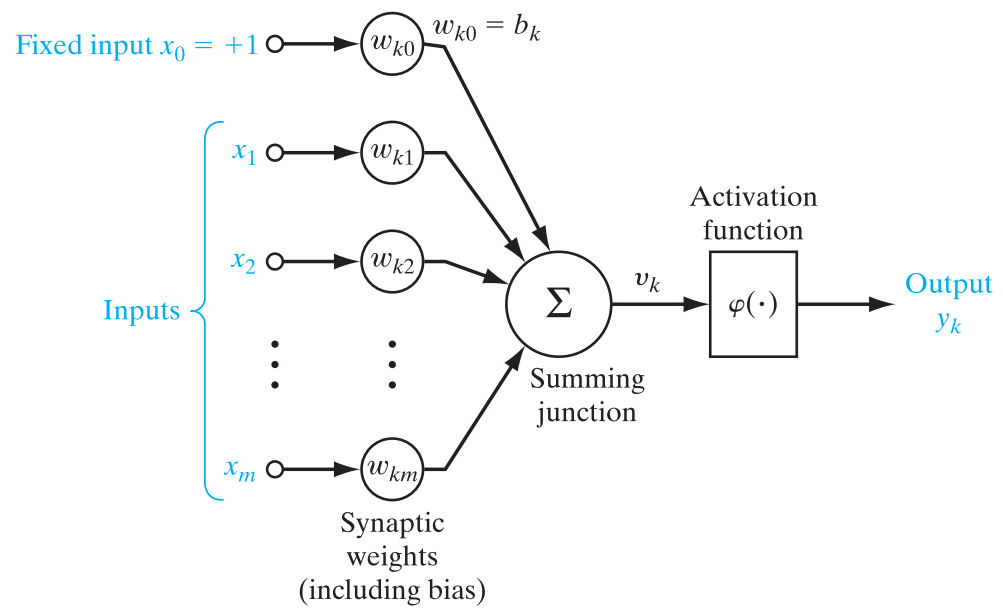• 突触（权值）$w_{ki}$ 每一个都由其权值或者强度作为特征。特别是，在连接到神经元$k$的突触$j$上的输入信号被乘以$k$的突触权值。
• 偏置$w_{k0}$, $x_{0}=+1$
   用来调节值域的。

• 加法器 是一个线性组合器，求输入信号与神经元相应突触权值的加权和，并与偏置求和。

$net_{k} = \sum_{i=1}^{m}x_{i}w_{ki}+w_{k0}=\sum_{i=0}^{m}x_{i}w_{ki}=w_{k}^{t}x$

# 3. 多层前馈神经网络(Multi-Layer Neural Network)

• 每层神经元与下一层神经元全互联
• 解决非线性可分问题——多层功能神经元
• 前馈（feedforward）并不意味着网络中信号不能后传，而是指网络拓扑结构上不存在环或回路。
• 输入层仅是接受输入，不进行函数处理，隐层与输出层包含功能神经元
• 隐层结点：由两层及两层以上结点组成的前馈网络中处于非输出层的结点称为隐层结点，一个只含一个隐含层的前馈网络称为双层前馈网络。含两个隐含层的称三层前馈网络。一般使用至多两个隐含层的网络。

• 函数信号
• 来自于输入端，到达输出端
• 误差信号
• 产生于输出端，反向传播

• 计算输出函数信号
• 输入信号和权值的连续非线性函数
• 计算梯度向量
• 误差曲面对于权值的梯度的估计

# 4. 学习

## 4.1. 最小均方算法(LMS)

• 定义代价函数(cost function)为均方误差 $\varepsilon(w)=\frac 1{2N}\sum_{n=1}^N e^2(n)$ 其中$e(n)$为时刻$n$测得的误差。 定义权值更新规则 $w\leftarrow w+\Delta w$ 基于最速下降法则 $\Delta w=-\frac {\partial \varepsilon (w)}{\partial w}=-\frac 1N\sum_{n=1}^N e( n)\frac {\partial e(n)}{\partial w}$ 要求激活函数可导。

• 定义代价函数(cost function)为瞬时误差的平方 $\varepsilon(w)=\frac 12 e^2(n)$ 在线学习（on-line） 称为串行方式、在线方式、随机方式 每个训练样本呈现之后，即进行权值更新. 训练集内的全部N个样本都呈现一次，称为一个回合(epoch)；

• 集中方式 $\varepsilon(w)=\frac 1{2N} \sum _{i=1}^N\sum _{k \in C}e_k^2(n)$

• 在线方式 $\varepsilon(w)=\frac 12 \sum _{k \in C}e_k^2(n)$

## 4.3. 误差逆传播算法(error BackPropagation)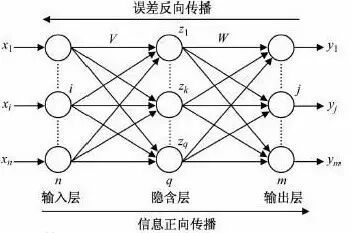### 4.3.1. 计算梯度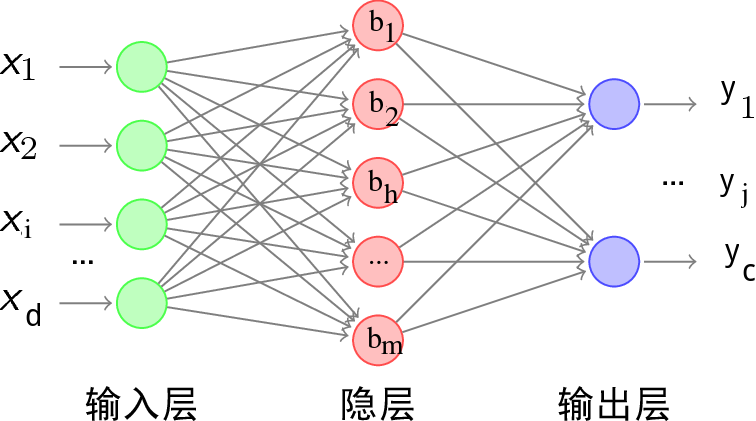• 隐层第$h$个神经元的阈值用$\gamma _h$表示，
• 输出层第$j$个神经元的阈值用$\theta_j$表示。
• 输入层第$i$个神经元与隐层第$h$个神经元之间的连接权为$v_{ih}$;
• 隐层第$h$个神经元与输出层第$j$个神经元之间的连接权为$w_{hj}$;
• 记隐层第$h$个神经元接收到的输入为$\alpha_h=\sum_{i=1}^dv_{ih}x_i$;
• 输出层第$j$个神经元接收到的输入为$\beta_j=\sum_{h=1}^mw_{hj}b_h$, 其中$b_h$为隐层第$h$个神经元的输出;
• 假设隐层和输出层神经元都使用Logistic函数： $P(t)=\frac 1{1+e^{-t}}$

# 5. 其他常见神经网络

## 5.6. Elman网络

### 5.6.1. Hebb学习

• Hebb规则： Hebb是神经生理学家, 在1949年提出一种假没，认为脑细胞中突触强度根据其感受到的经验改变。他因此提出这种突触强度的改变与神经在触发前后突触之间的相关成正比。由于在Hopfield模型中采用的联接规则与Hebb的修改相比，因而在人工神经之网络中广泛使用的规则以Hebb冠名。这种规则除了在Hopfield模型中使用外，在非监督学习方法的人工神经元网络中广泛采用。
• 非监督Hebb学习方法：人工神经元网络也用来分析数据集内的一些规律，如方差分析，主分量分析等，由于其训练方法采用了Hebb规则，因而称为非监督Hebb学习方法。